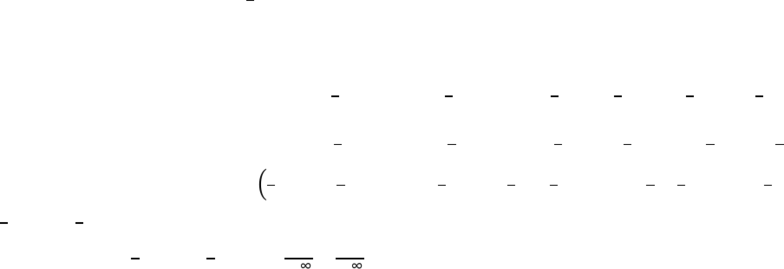Class Notes (1,100,000)
CA (630,000)
UTSG (50,000)
MAT (4,000)
MAT136H1 (900)
all (200)
Lecture

7.8 Improper Integrals Question #4 (Medium)

Department
Mathematics
Course Code
MAT136H1
Professor
all

Page:
of 17.8 Integration Techniques
Improper Integrals
Question #4 (Medium): Convergent Improper Integral
Strategy
If both sides of the integral are infinity, then split the integral with respect to any number, but a nice
number would be beneficial in saving time for computation, like or . Then add limit with variable ,
since is already taken. Then solve the integral disregarding the limit, then afterward apply the value
that variable t approaches to determine convergence or divergence. If convergent, as limit value is
plugged in, it will figure itself out.
Sample Question
Determine if the integral is convergent or divergent. If convergent, evaluate the integral.


Solution
Since both sides approach infinity, split the integral. Since there is with no denomination, would be
good. Then: 
 
 
. Add the limit: 
 

 
. Then solve the function in the integral disregarding the limits for now.
The function follows after chain rule, so if comfortable solve as it is. If using substitution, let   ,
then   , then 
. The interval has to align with the substitution. So when   ,
   , and when  ,  . Because the integral is cut symmetrically, same info can be used
for both integrals.
Taking the first integral: 


 



And the second integral:


 

  

Add the two with the limit: 


 
 

 




They cancel out:



    
Therefore, the integral converges to 
  